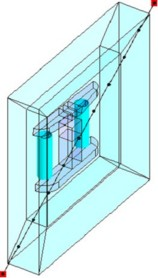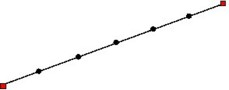## Definition

A path is a line support that includes sections and on which the user can evaluate a spatial quantity.

## Use

A path is used to plot 2D curves (see 2D curve (about)).

## Geometric shapes

The sections of path can be as follows:

• rectilinear (straight segment)
• curvilinear (arc of circle, helicoidal line)

## Path and infinite box

The spatial support of the path type can be extended beyond the bounded study domain.## Mesh

The mesh of a path is based on a user-defined discretization: computation points are regularly spaced on the line of the support. It can be defined by:

• a number of intervals
• a number of points
• a distance between two points (mm)## Types of sections

Types of sections are as follows:

Type of sections Shape of path Definition
section defined by 2 points

straight segment /

arc of circle

helicoidal line

2 points*

(graphical or numerical capture)

arc defined by 3 points arc of circle

3 points*

(graphical or numerical capture)

arc defined by center, radius and angles arc of circle

center coordinates,

starting angle and ending angle

parameterized path

straight segment /

arc of circle

helicoidal line

parameter functions
existing Line entity all shapes of lines graphical selection of the existing line
Note: * To capture points, refer to § Computation point.

## Section defined by 2 points

There are many options provided to the user in case of the section defined by 2 points and these points are defined on cylindrical faces. This is presented in the table below.

Option Description
StraightLine2Pt Straight segmentShortOnFace… Shortest path between two points on the cylindrical face
LongOnFace… Longest path between two points on the cylindrical face

## Parameter setting

The user can use geometric parameters to define the section by the numerical mode.

## Path and motion

Within a study with kinematic coupling, there is a motion of the moving part of the device.

The path belonging to the moving part can be defined in motion with the moving part or remaining fixed during the movement.

The path can thus be defined as:

• a mobile path if it is associated to a mobile mechanical set
• a fixed path if it is associated to a fixed mechanical set